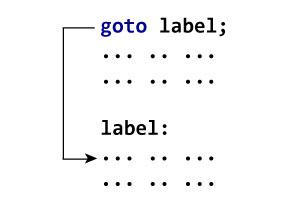# Explore hunderds of solved example topic wise.

## Jump Statements

### Jump Statements

Jump statements are used to change the normal flow of the program. It is used to jumps the control from one place to another.

There are 5 jump statements in C programming.

1. break
2. continue
3. goto
4. exit()
5. return

### break statement in C

The break statement is used to jump the control out of the current block. It only works with switch and loop.

See the example below to understand the break keyword.

#### break statement example

```for(i = 1; i <= 5; i++) {
if(i == 3) {
break;
}
printf("%d ",i);
}
```

#### Output

```1 2
```

As shown in the above example, the break statement executes when the value of i will be 3 and jumps the control out of the current block. so the output will be 1 and 2.

#### break statement with a nested loop

```for(i = 1; i <= 5; i++) {
for(j = 1; j <= 5; j++) {
if(j > i) {
break;
}
printf("%d ",i);
}
printf("\n");
}
```

#### Output

```1
1 2
1 2 3
1 2 3 4
1 2 3 4 5
```

As in the above example, the inner loop terminates as soon as j > i returns true. Note in the above example, the break statement only jumps the control out of the inner loop. Trace the above code-snippet to understand the output.

Note: We have already learned the use of break keyword with switch statements. Click here to review how to use the break keyword with the switch statement.

### continue statement in C

continue statement repeat the loop for the next round and skip all the statements written after it. continue statement only works with loop.

Read the following example to understand the continue statement.

#### Continue statement example

```for(i = 1; i <= 5; i++) {
if(i == 3) {
continue;
}
printf("%d ",i);
}
```

#### Output

```1 2 4 5
```

As shown in the above example, which i == 3 returns true loop immediately jumps to the increment and skip the printing statement for that iteration. So from 1 to 5 printing, 3 will be skipped and output will be 1 2 4 5.

#### Continue with nested loop example

```for(i = 1; i <= 5; i++) {
for(j = 1; j <= 5; j++) {
if(i == j) {
continue;
}
printf("%d ",j);
}
printf("\n");
}
```

#### Output

```2 3 4 5
1 3 4 5
1 2 4 5
1 2 3 4
1 2 3 4
```

If you trace the above program you will find that inner loop repeated when i == j returns true, so the above code snippet prints 1 to 5 in each line, but by using continue statement when i==j returns true printing skipped and generates above output.

### goto statement in C

The goto statement is used to jumps the control from one place to another place in your program. To mark a place in the program labels are used as shown in the following figure.Read the following example to understand the use of the goto statement.

#### goto statement example

```#include "stdio.h"

int main() {
int n;
printf("Enter any number: ");
scanf("%d",&n);

if(n >= 0) {
goto pos;
}else {
goto neg;
}

pos: //label
printf("%d is +ve",n);
return 0;
neg: //label
printf("%d is -ve",n);
return 0;
}
```
1. As shown in the above example, goto statements jump the control to the defined label.
2. It is not a good programming practice to use the goto statement because it creates extra overheads in the background.
3. It can jump the control out of the scope.
4. It can create infinite loops.
5. The use of goto can be easily avoided by using break, continue, and functions.

### exit() function in C

exit() is a function defined in stdlib.h. It terminates the whole program while terminating the program exit status should be returned to OS. The syntax of the exit() function is as follows...

```void exit(int status)
```

status value can be EXIT_SUCCESS and EXIT_FAILURE represented by 0 and 1 respectively. These two constants are defined in stdlib.h

### exit() example in C

```#include "stdio.h"
#include "stdlib.h"
int main() {
int age;

scanf("%d",&age);

if(age < 0) {
printf("Invalid age");
exit(EXIT_FAILURE); // exit(1)
}